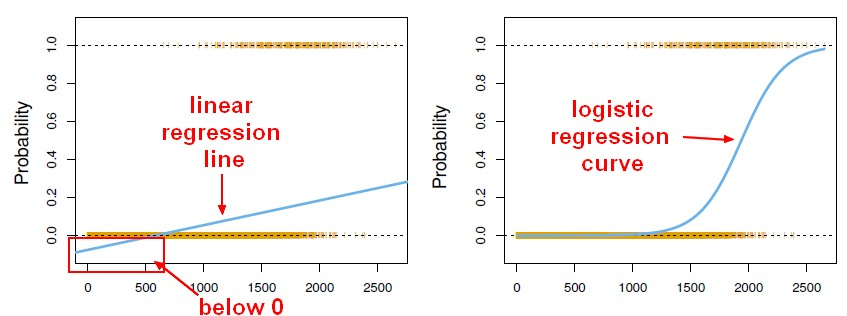# Statistics - Maximum likelihood

Maximum likelihood was introduced by Ronald Fisher back in the 1920s.

Since each observation is meant to be independent of each other one, the probability of observed data is the probability of the observed class (for a binary class: 0's and 1's)

$$\begin{array}{rrl} likelihood(B_0,B_1) & = & \prod_{i:y_i=1}p(X_i) \prod_{i:y_i=0}(1-p(X_i)) \end{array}$$

where:

• $\displaystyle \prod_{i:y_i=1}$ is the probability of i when y=1.

The idea of maximum likelihood is pick the parameters to make that probability as large as possible.

The probability is represented by a function called the “log-likelihood function”.

Recommended PagesMachine Learning - (Univariate|Simple) Logistic regression

A Simple Logistic regression is a Logistic regression with only one parameters. For the generalization (ie with more than one parameter), see Logistic regression comes from the fact that linear regression...Statistics - Akaike information criterion (AIC)

AIC stands for Akaike Information Criterion. Akaike is the name of the guy who came up with this idea. AIC is a quantity that we can calculate for many different model types, not just linear models,...